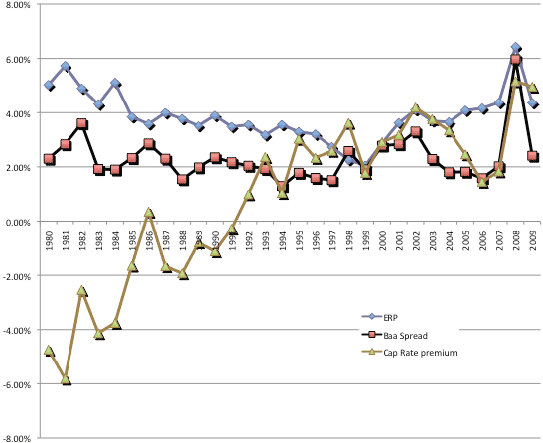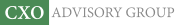Objective research to aid investing decisions

#### Value Investing Strategy (Strategy Overview)

Allocations for October 2023 (Final)
Cash TLT LQD SPY

#### Momentum Investing Strategy (Strategy Overview)

Allocations for October 2023 (Final)
1st ETF 2nd ETF 3rd ETF

# An Era of Unstable Risk Premiums?

| | Posted in: Big Ideas, Equity Premium, Strategic Allocation

How stable are risk premiums? How should investors respond to instabilities? In his August 2010 paper entitled “A New ‘Risky’ World Order: Unstable Risk Premiums: Implications for Practice”, Aswath Damodaran presents approaches for estimating equity, bond and real asset risk premiums that are imprecise, unstable and linked across markets. He also explores the implications of dynamic, linked premiums for asset allocation, market timing and asset valuation. Using long-run data for all three asset classes, he concludes that:

• Asset class risk premiums are functions of:
• Investor risk aversion (in turn a function of investor age and preference for current versus future consumption)
• Economic risk (health and predictability of the overall economy)
• Information (quantity and quality)
• Catastrophic risk (war, depression, hyperinflation)
• Investor irrationality (money illusion, narrow framing)
• To some degree, changes in these variables link risk premiums for different asset classes by making them move in the same direction.
• Evidence from historical data indicates that estimates of asset class risk premiums (see the chart below):
• Are imprecise when derived from historical returns (even very long series).
• Are unstable over both short and long horizons when derived from implied returns of current prices and expected cash flows.
• Do not revert to predictable means over predictable intervals.
• Are linked rather than insulated from each other (via some of the above variables and competition for investment funds).
• Investors should:
• In allocating across asset classes, track differences between dynamic estimates of risk premiums for different asset classes relative to historical gaps (for example, track the ratio of the estimated equity risk premium to the bond default spread).
• In allocating across asset classes, compare risk-adjusted dynamic estimates of risk premiums (for example, compare ratios of equity risk premium to realized volatility of returns for different country stock markets).
• In timing an asset class, compare a dynamic estimate of the risk premium for that class (based on implied returns of current prices and expected cash flows) to historical values of the dynamic estimate.
• Be cautious in assuming mean reversion.

The following chart, taken from the paper, compares dynamic estimates of the risk premiums for U.S. equities, corporate bonds and real estate over the past three decades. Risk premium estimates derive from:

• Equity (ERP): the return implied by current prices and estimated future cash flows.
• Bonds (Baa Spread): the default spread, which is the difference between corporate Baa bond yield and 10-year Treasury note yield.
• Real estate (Cap Rate premium): the capitalization rate, which is the discount rate applied to a real estate property’s earnings to arrive at an estimate of value.

The equity risk premium and the default spread generally track, though there are relatively short periods when they diverge. With the advent of real estate securitization (mortgage-backed securities) in the 1990s, the real estate capitalization rate links with the other two premiums. Investors in any one of these markets should track risk premiums in the other markets to assess relative valuations and allocate assets accordingly.In summary, investors should assess and compare asset classes based on dynamic estimates of respective risk premiums (implied returns derived from current prices and estimated cash flows) to guide asset class allocation and market timing.

It seems possible that, at times, even the most attractive asset class might have an historically unattractive risk premium.